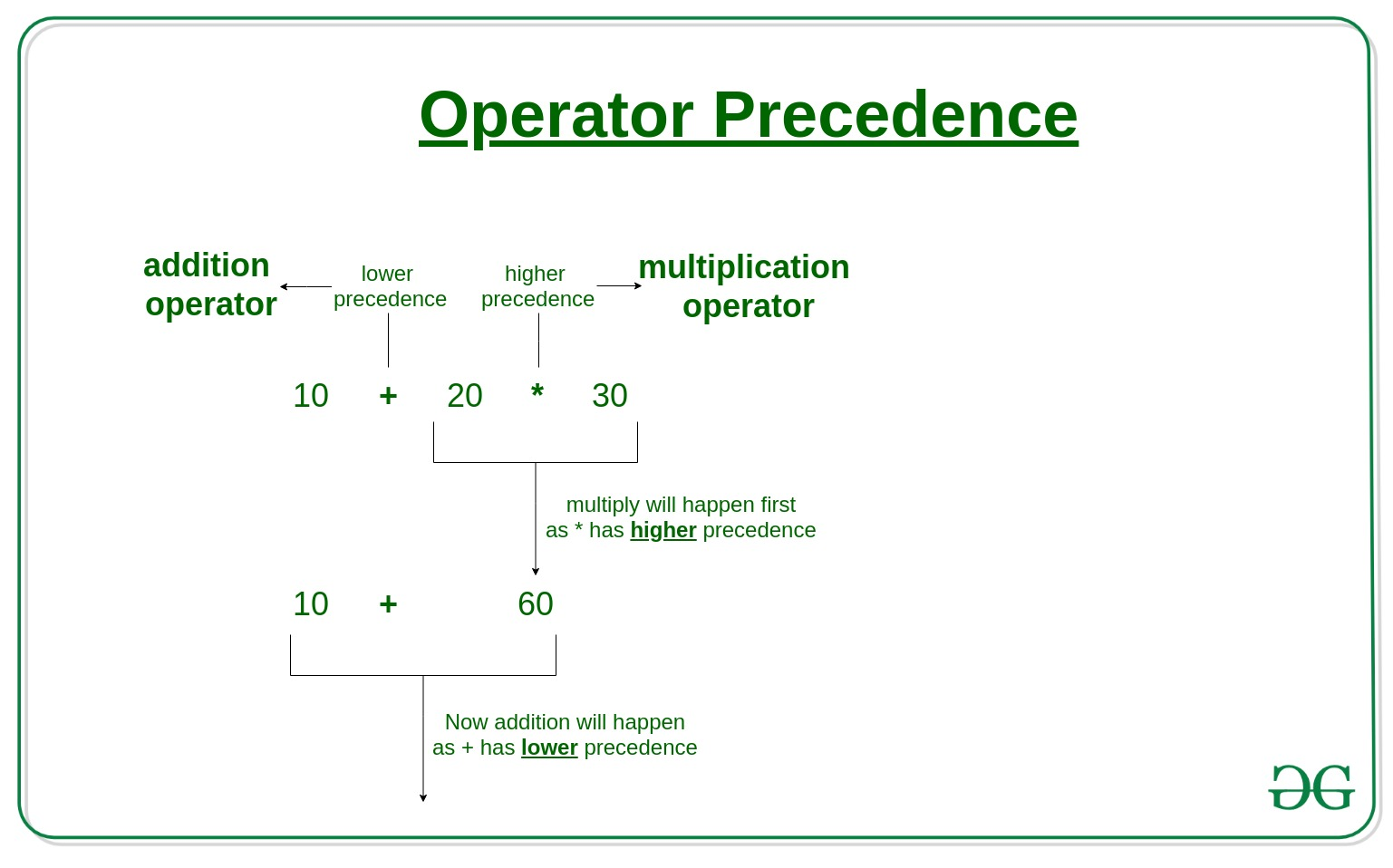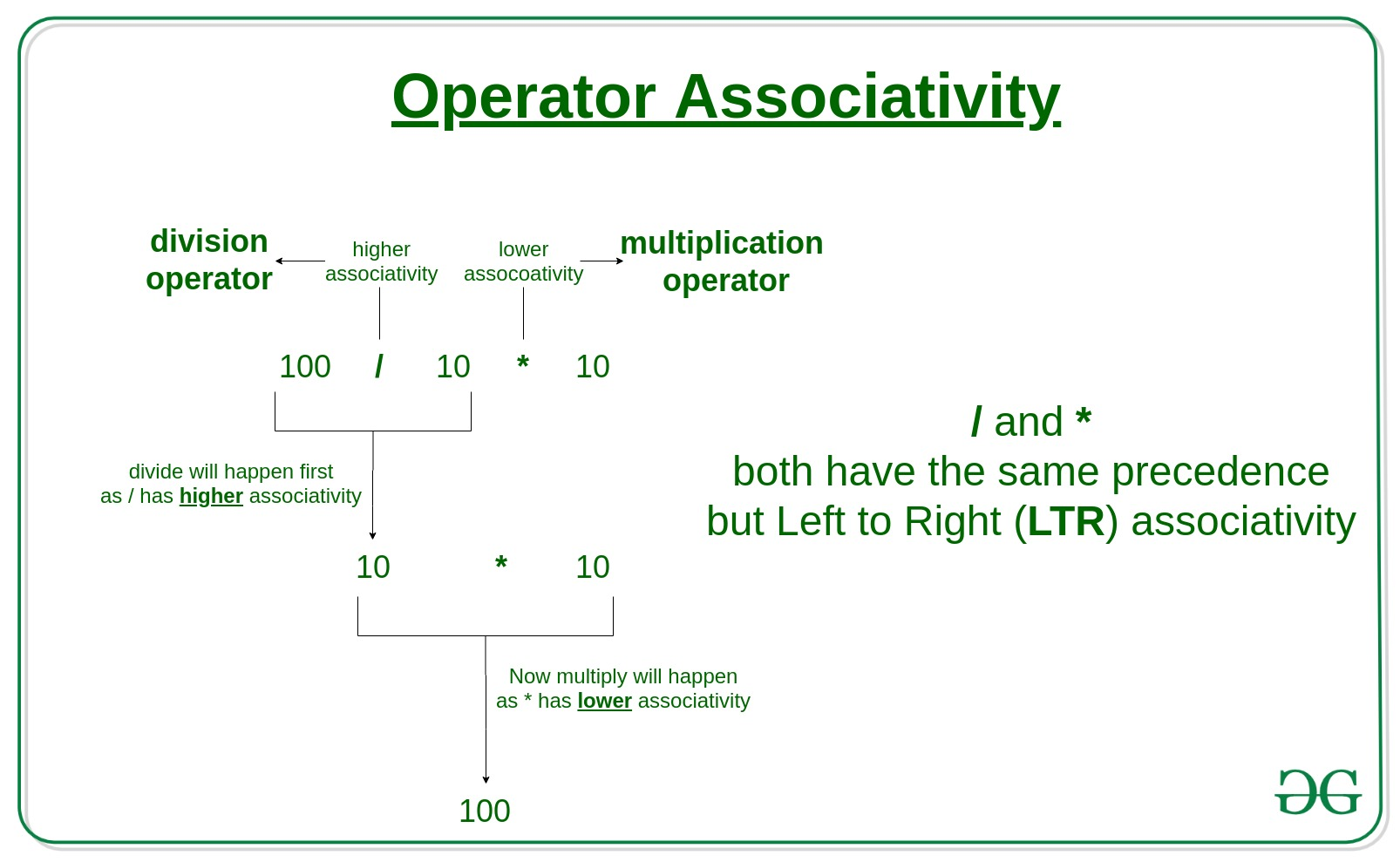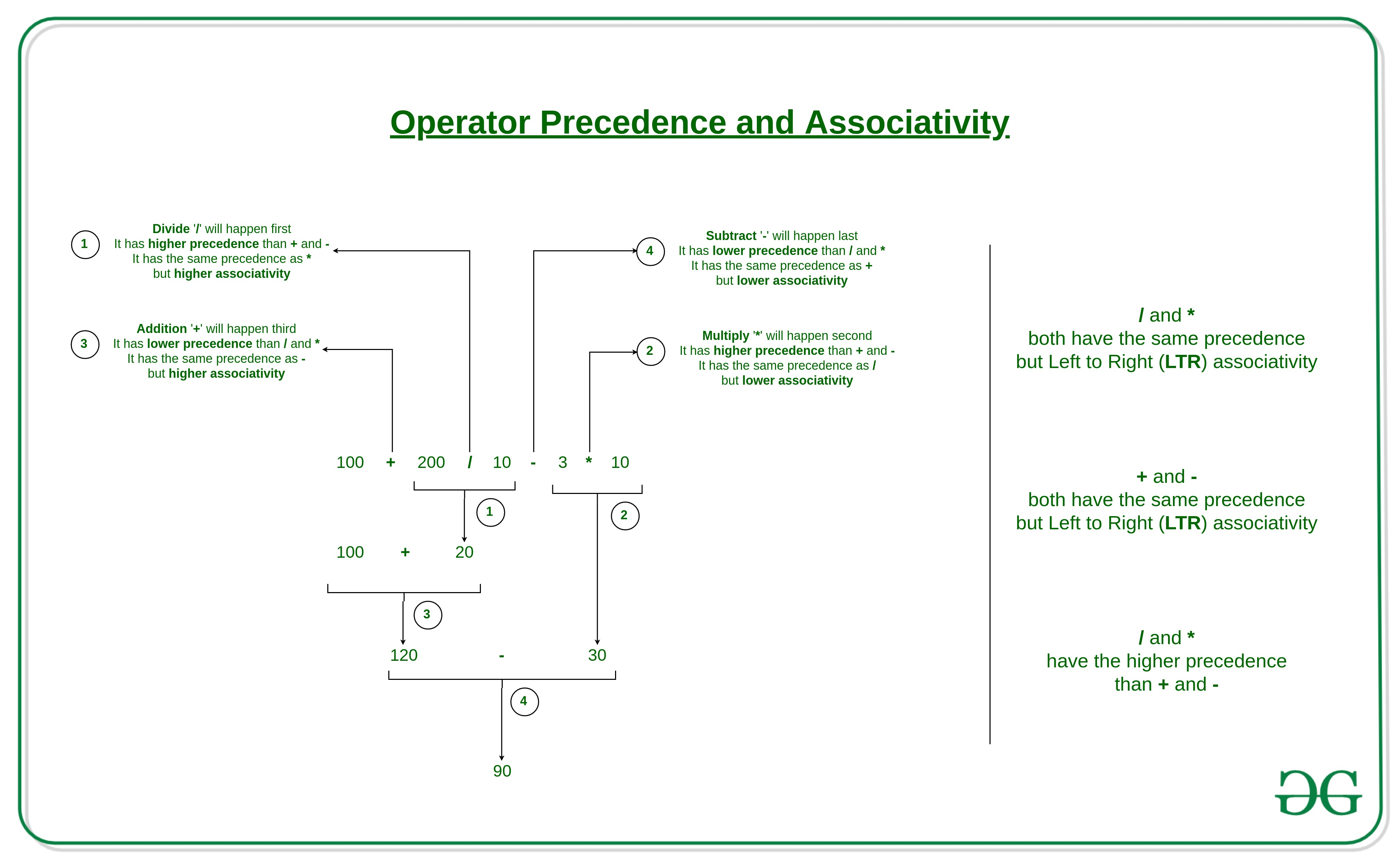Related Articles
Operator Precedence and Associativity in C
• Difficulty Level : Easy
• Last Updated : 08 Jul, 2019

Operator precedence determines which operator is performed first in an expression with more than one operators with different precedence.

For example: Solve

`10 + 20 * 30````10 + 20 * 30 is calculated as 10 + (20 * 30)
and not as (10 + 20) * 30```

Operators Associativity is used when two operators of same precedence appear in an expression. Associativity can be either Left to Right or Right to Left.

For example: ‘*’ and ‘/’ have same precedence and their associativity is Left to Right, so the expression “100 / 10 * 10” is treated as “(100 / 10) * 10”.Operators Precedence and Associativity are two characteristics of operators that determine the evaluation order of sub-expressions in absence of brackets

For example: Solve

`100 + 200 / 10 - 3 * 10`1) Associativity is only used when there are two or more operators of same precedence.
The point to note is associativity doesn’t define the order in which operands of a single operator are evaluated. For example, consider the following program, associativity of the + operator is left to right, but it doesn’t mean f1() is always called before f2(). The output of the following program is in-fact compiler dependent. See this for details.

 `// Associativity is not used in the below program.``// Output is compiler dependent.`` ` `#include `` ` `int` `x = 0;``int` `f1()``{``    ``x = 5;``    ``return` `x;``}``int` `f2() ``{``    ``x = 10;``    ``return` `x;``}``int` `main()``{``    ``int` `p = f1() + f2();``    ``printf``(``"%d "``, x);``    ``return` `0;``}`

2) All operators with the same precedence have same associativity
This is necessary, otherwise, there won’t be any way for the compiler to decide evaluation order of expressions which have two operators of same precedence and different associativity. For example + and – have the same associativity.

3) Precedence and associativity of postfix ++ and prefix ++ are different
Precedence of postfix ++ is more than prefix ++, their associativity is also different. Associativity of postfix ++ is left to right and associativity of prefix ++ is right to left. See this for examples.

4) Comma has the least precedence among all operators and should be used carefully For example consider the following program, the output is 1. See this and this for more details.

 `#include ``int` `main()``{``    ``int` `a;``    ``a = 1, 2, 3; ``// Evaluated as (a = 1), 2, 3``    ``printf``(``"%d"``, a);``    ``return` `0;``}`

5) There is no chaining of comparison operators in C
In Python, expression like “c > b > a” is treated as “c > b and b > a”, but this type of chaining doesn’t happen in C. For example consider the  following program. The output of following program is “FALSE”.

 `#include ``int` `main()``{``    ``int` `a = 10, b = 20, c = 30;`` ` `    ``// (c > b > a) is treated as ((c  > b) > a), associativity of '>'``    ``// is left to right. Therefore the value becomes ((30 > 20) > 10)``    ``// which becomes (1 > 20)``    ``if` `(c > b > a)``        ``printf``(``"TRUE"``);``    ``else``        ``printf``(``"FALSE"``);``    ``return` `0;``}`

Please see the following precedence and associativity table for reference.

Operator

Description

Associativity

( )
[ ]
.
->
++ —
Parentheses (function call) (see Note 1)
Brackets (array subscript)
Member selection via object name
Member selection via pointer
Postfix increment/decrement (see Note 2)

left-to-right

++ —
+ –
! ~
(type)
*
&
sizeof
Prefix increment/decrement
Unary plus/minus
Logical negation/bitwise complement
Cast (convert value to temporary value of type)
Dereference
Address (of operand)
Determine size in bytes on this implementation
right-to-left
*  /  %Multiplication/division/modulusleft-to-right
+  –Addition/subtractionleft-to-right
<<  >>Bitwise shift left, Bitwise shift rightleft-to-right
<  <=
>  >=
Relational less than/less than or equal to
Relational greater than/greater  than or equal to
left-to-right
==  !=Relational is equal to/is not equal toleft-to-right
&Bitwise ANDleft-to-right
^Bitwise exclusive ORleft-to-right
|Bitwise inclusive ORleft-to-right
&&Logical ANDleft-to-right
| |Logical ORleft-to-right
? :Ternary conditionalright-to-left
=
+=  -=
*=  /=
%=  &=
^=  |=
<<=  >>=
Assignment
Addition/subtraction assignment
Multiplication/division assignment
Modulus/bitwise AND assignment
Bitwise exclusive/inclusive OR assignment
Bitwise shift left/right assignment
right-to-left

,

Comma (separate expressions)left-to-right

It is good to know precedence and associativity rules, but the best thing is to use brackets, especially for less commonly used operators (operators other than +, -, *.. etc). Brackets increase the readability of the code as the reader doesn’t have to see the table to find out the order.

Please write comments if you find anything incorrect, or you want to share more information about the topic discussed above

Attention reader! Don’t stop learning now. Get hold of all the important DSA concepts with the DSA Self Paced Course at a student-friendly price and become industry ready.

My Personal Notes arrow_drop_up